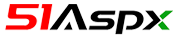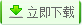| | 帮助?

[免费版 Free] WebForm,下载次数：418 次 | 关键字： 考试 办公 高校 竞赛 企事业单位## 源码目录树

;
```当前路径：vendor/sebastian/diff/tests/LongestCommonSubsequenceTest.php
<?php declare(strict_types=1);
/*
* This file is part of sebastian/diff.
*
* (c) Sebastian Bergmann <sebastian@phpunit.de>
*
* file that was distributed with this source code.
*/

namespace SebastianBergmann\Diff;

use PHPUnit\Framework\TestCase;

/**
* @coversNothing
*/
abstract class LongestCommonSubsequenceTest extends TestCase
{
/**
* @var LongestCommonSubsequenceCalculator
*/
private \$implementation;

/**
* @var string
*/
private \$memoryLimit;

/**
* @var int[]
*/
private \$stress_sizes = [1, 2, 3, 100, 500, 1000, 2000];

protected function setUp(): void
{
\$this->memoryLimit = \ini_get('memory_limit');
\ini_set('memory_limit', '256M');

\$this->implementation = \$this->createImplementation();
}

protected function tearDown(): void
{
\ini_set('memory_limit', \$this->memoryLimit);
}

public function testBothEmpty(): void
{
\$from   = [];
\$to     = [];
\$common = \$this->implementation->calculate(\$from, \$to);

\$this->assertSame([], \$common);
}

public function testIsStrictComparison(): void
{
\$from = [
false, 0, 0.0, '', null, [],
true, 1, 1.0, 'foo', ['foo', 'bar'], ['foo' => 'bar'],
];
\$to     = \$from;
\$common = \$this->implementation->calculate(\$from, \$to);

\$this->assertSame(\$from, \$common);

\$to = [
false, false, false, false, false, false,
true, true, true, true, true, true,
];

\$expected = [
false,
true,
];

\$common = \$this->implementation->calculate(\$from, \$to);

\$this->assertSame(\$expected, \$common);
}

public function testEqualSequences(): void
{
foreach (\$this->stress_sizes as \$size) {
\$range  = \range(1, \$size);
\$from   = \$range;
\$to     = \$range;
\$common = \$this->implementation->calculate(\$from, \$to);

\$this->assertSame(\$range, \$common);
}
}

public function testDistinctSequences(): void
{
\$from   = ['A'];
\$to     = ['B'];
\$common = \$this->implementation->calculate(\$from, \$to);
\$this->assertSame([], \$common);

\$from   = ['A', 'B', 'C'];
\$to     = ['D', 'E', 'F'];
\$common = \$this->implementation->calculate(\$from, \$to);
\$this->assertSame([], \$common);

foreach (\$this->stress_sizes as \$size) {
\$from   = \range(1, \$size);
\$to     = \range(\$size + 1, \$size * 2);
\$common = \$this->implementation->calculate(\$from, \$to);
\$this->assertSame([], \$common);
}
}

public function testCommonSubsequence(): void
{
\$from     = ['A',      'C',      'E', 'F', 'G'];
\$to       = ['A', 'B',      'D', 'E',           'H'];
\$expected = ['A',                'E'];
\$common   = \$this->implementation->calculate(\$from, \$to);
\$this->assertSame(\$expected, \$common);

\$from     = ['A',      'C',      'E', 'F', 'G'];
\$to       = ['B', 'C', 'D', 'E', 'F',      'H'];
\$expected = ['C',                'E', 'F'];
\$common   = \$this->implementation->calculate(\$from, \$to);
\$this->assertSame(\$expected, \$common);

foreach (\$this->stress_sizes as \$size) {
\$from     = \$size < 2 ?  : \range(1, \$size + 1, 2);
\$to       = \$size < 3 ?  : \range(1, \$size + 1, 3);
\$expected = \$size < 6 ?  : \range(1, \$size + 1, 6);
\$common   = \$this->implementation->calculate(\$from, \$to);

\$this->assertSame(\$expected, \$common);
}
}

public function testSingleElementSubsequenceAtStart(): void
{
foreach (\$this->stress_sizes as \$size) {
\$from   = \range(1, \$size);
\$to     = \array_slice(\$from, 0, 1);
\$common = \$this->implementation->calculate(\$from, \$to);

\$this->assertSame(\$to, \$common);
}
}

public function testSingleElementSubsequenceAtMiddle(): void
{
foreach (\$this->stress_sizes as \$size) {
\$from   = \range(1, \$size);
\$to     = \array_slice(\$from, (int) (\$size / 2), 1);
\$common = \$this->implementation->calculate(\$from, \$to);

\$this->assertSame(\$to, \$common);
}
}

public function testSingleElementSubsequenceAtEnd(): void
{
foreach (\$this->stress_sizes as \$size) {
\$from   = \range(1, \$size);
\$to     = \array_slice(\$from, \$size - 1, 1);
\$common = \$this->implementation->calculate(\$from, \$to);

\$this->assertSame(\$to, \$common);
}
}

public function testReversedSequences(): void
{
\$from     = ['A', 'B'];
\$to       = ['B', 'A'];
\$expected = ['A'];
\$common   = \$this->implementation->calculate(\$from, \$to);
\$this->assertSame(\$expected, \$common);

foreach (\$this->stress_sizes as \$size) {
\$from   = \range(1, \$size);
\$to     = \array_reverse(\$from);
\$common = \$this->implementation->calculate(\$from, \$to);

\$this->assertSame(, \$common);
}
}

public function testStrictTypeCalculate(): void
{
\$diff = \$this->implementation->calculate(['5'], ['05']);

\$this->assertIsArray(\$diff);
\$this->assertCount(0, \$diff);
}

/**
* @return LongestCommonSubsequenceCalculator
*/
abstract protected function createImplementation(): LongestCommonSubsequenceCalculator;
}
```

Floor 5th,Daheng Building,Zhongguancun,Beijing,China,100080